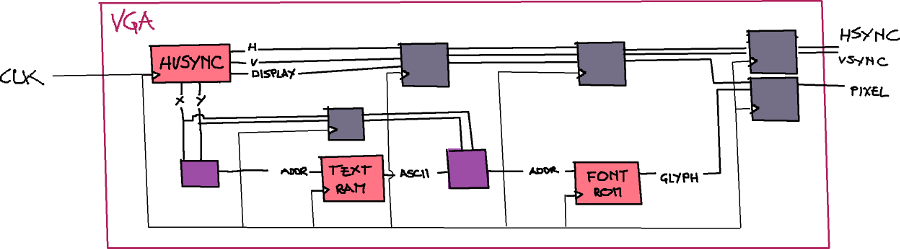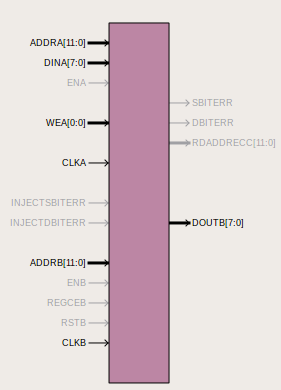# Implementing Text mode for a VGA controller in Verilog

After having written about implementing a VGA controller in Verilog I wanted to improve it with a new functionality: the Text mode.

The Text mode is described in an excellent way by Wikipedia

Text mode is a computer display mode in which content is internally represented
on a computer screen in terms of characters rather than individual pixels.
Typically, the screen consists of a uniform rectangular grid of character cells,
each of which contains one of the characters of a character set


It's not a difficult project but it's worth making. I start laying out the diagram of the controller:The purple boxes are combinatorial logic realizing the translation between screen's coordinates and the memories addresses, the grey boxes instead are flip-flops intended to sync signals between the different internal blocks of the module: this will be explained later.

With respect to the previous VGA controller here we have two new elements: the ROM with the predefined glyph and the Text RAM containing the characters I want to display on screen. These are memories.

The hvsync_generator is pratically unchanged from the version of the previous post.

### Memory

A memory is a device capable of storing and accessing data (you know, memory); usually are characterized by its address width and output width.

Since I'm using a spartan-6 FPGA I have access to some primitive useful to generate memories using the Core generator (here a guide: Spartan-6 FPGA Block RAM Resources).

For the ROM I have the following diagramthe clock (CLKA) signal is obvious, the other are the address line (ADDRA) and the output (DOUTA); the width of these lines are determined by the organization of the memory you need.

In my case I need the ROM to contain the data necessary to draw the pixel of a single character: I'll use 128 ASCII characters and each one will be represented with a 8x16 grid; in this way I need 16384 bits. Since I want to access the bits individually I configure the memory to be 16Kx1Different story is the RAM: I need a read and write memory device that will contain an ASCII character index for each one of the block in which the screen is divided; being the size of each character be 8x16 we have, for a resolution of 640x480, 80 columns and 30 rows.

This means the we need 2400 bytes, i.e. the memory organized as 2400x8.Since I need to write, there are two signal lines for that (DINA and ADDRB) that in this implementation will remain unused.

### Implementation

How we translate the screen's coordinates into text memory address and glyph memory address?

The idea here is that the value of the pixel is decided calculating in which $$column, row$$ belongs: this can be determined using its coordinates $$(x, y)$$ with a division respectively by 8 and 16; in digital logic is simpler to take only the most significant bits of these signals:

// (column, row) = (x / 8, y / 16)
assign column = x[9:3];
assign row = y[8:4];


and at this point we can calculate the address into the text memory in order to extract the ASCII character we need

assign text_address = column + (row * 80);

text_memory tm(
.clka(clk), // input clka
.wea(wea), // input [0 : 0] wea
.dina(dina), // input [7 : 0] dina
.clkb(clk), // input clkb
.doutb(text_value) // output [7 : 0] doutb
);


The final value of the pixel is obtained using the modulo of the pixel's coordinates as index for the glyph into the ROM

// here we get the remainder
always @(posedge clk) begin
glyph_x <= x[2:0];
glyph_y <= y[3:0];
end
// text_value * 128 + glyph_x + (glyph_y * 8)
assign glyph_address = (text_value << 7) + glyph_x + (glyph_y << 3);

blk_mem_gen_v7_3 glyph_rom(
.clka(clk), // input clka
.douta(pixel) // output [0 : 0] douta
);


Also here the multiplications by power of two are implemented with simpler left shifts.

### Glyph and ROM

To display characters I need obviously some of them; for this project I used the Unicode VGA font. This font is distributed using the BDF format.

This format is, simply put, a text format that descibes the geometry and the points forming the glyph

STARTCHAR space
ENCODING 32
SWIDTH 480 0
DWIDTH 8 0
BBX 8 16 0 -4
BITMAP
00
00
00
00
00
00
00
00
00
00
00
00
00
00
00
00
ENDCHAR


In order to be used into the Xilinx IDE, the glyph must be converted into a COE format, that is simply a binary representation of the words into the memory.

Since I didn't know any program that does that, I modified an existing program that converts BDF glyphs into C header files and added the option to dump in the needed format: the repo is here bdf2c but don't look at the code, is very ugly :P.

As final step I dumped the characters needed

\$ cat fpga/mojo/VGAGlyph/font/uni_vga/u_vga16.bdf  | /opt/bdf2c/bdf2c -z glyph.coe -b


and synthetized with the Xilinx tools the design.

### Sync

The first attempt for this controller had some issues with timing, something like the situation described here.

Roughly speaking, every memory block needs one clock cycle to output the value it's storing, since we have two memories in series we need two clock cycles to obtain the pixel values to output on the screen. So the pixel data and the hsync and vsync signals are out of synch causing the character to be not aligned to the side of the screen.

Another connection that needs an explicit synchronization is the combinational logic that translates the screen's coordinates for the glyph ROM: as just said, only after one clock cycle the ASCII value is output from the text memory, so using directly the glyph coordinates derived from the screen ones gives you the wrong glyph data; this is the reason for the sequential always@(posedge clk) instruction.

I want to stress here that the approach chosen is to delay the signals out of synch in order to align it; this is accomplished using some flip flops.

When I started thinking to this problem I came up with different options in order to solve it

1. pass the $$x, y$$ coordinates decremented of two
2. pass a clock twice as fast as the one for the hsync and vsync
3. read the character memory only once every eigth clock cycles

but thanks to a chapter of "FPGA prototyping by Verilog examples" I realized that is simpler to "slow down" the signals; it's not probably a solution for all the use cases, but this particular one, where the signal data is generated continuosly, I think is a good situation.

By the way, the implementation of the delay uses the following block of code

always@(posedge clk) begin
hsync_delayed1 <= hsync_out_original;
hsync_delayed2 <= hsync_delayed1;
hsync_delayed3 <= hsync_delayed2;

vsync_delayed1 <= vsync_out_original;
vsync_delayed2 <= vsync_delayed1;
vsync_delayed3 <= vsync_delayed2;

end

assign hsync_out = hsync_delayed3;
assign vsync_out = vsync_delayed3;


inside the VGA module.

### Summary

My advice, when some issue arises from a design, is to draw explicitely a diagram of the different blocks composing your system like my awesome™ hand-drawn scheme.

In this way you can see clearly the timing and connections. The best way however is to create a reliable testbench to test the design, but this is a topic for another day.

At the end it seems to work just fine :)If you are interested in the project, the source code can be found on github.